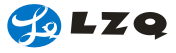229
 Calculation formula of milling machining
 ◆.Cutting speed (VC)
 Cutting speed Circumference ratio Diameter of mill (mm) Rotation speed of spindle
* Dividing by 1000 is to convert mm into m. ((Example) Rotation speed of spindle is 350min-1, diameter of mill isφ125. Calculate cutting speed.

（(Answer) Integrate ?3.14, D1=125, n=350 in the formula,

From this, we get cutting speed is 137.4m/min.

 ◆. Feed per tooth
 Feed per tooth Feed speed of worktable per minute Rotation speed of spindle (feed per revolution fr=z×fz ) Number of tooth
*Minor cutting edge angle (Example) Rotation speed of spindle is 500 min-1, number of mill's teeth is 10,
feed speed of worktable is 500mm/min, calculate feed per tooth.

we get feed per tooth is 0.1mm/tooth

 ◆. Feed speed of worktable (vf)
 Feed speed of worktable per minute Feed per tooth Rotation speed of spindle Number of tooth

((Example) Feed per tooth is 0.1mm/tooth, number of mill's tooth is 10,
feed and rotation speed of spindle is 500 min-1, calculate feed speed of worktable.

We get feed speed per tooth is 500mm/min.

 ◆. Machining time (Tc)
 Machining time Feed speed of worktable per minute Total feed length of worktable (workpiece length 1 + diameter of mill:D1)

(Example) Flat cast iron (FC200) sheet is 100mm wide, 300mm long, diameter of mill isφ200,
the number of mill's teeth is 16, cutting speed is 125m/min, feed per tooth is 0.25mm
Calculate machining time required (rotation speed of spindle is 200min-1）
(Answer) firstly calculate feed speed of worktable per minute vf=0.25*16*200=800mm/min
Then calculate total feed length of worktable L=300+200=500mm, integrate them in the formula,

We get the conclusion that it needs about 37.5s.-216-